What follows is an example of an exploratory analysis of the Kennebec River (Maine, USA) discharge rates over time. This analysis is conducted in the spirit of John Tukey in that it leverages both re-expression and robustness in teasing out trends in the data.

The data were pulled from the USGS. The gage station site id is `01049500` and is located in Gardiner , Maine (USA). The data span the years 1976 through 2020.``````library(dplyr)
library(lubridate)
library(ggplot2)

## Initial plot

An initial plot of the discharge rate (in ft3/s) follows.

``````ggplot(dat, aes(Date, Discharge)) + geom_line() +
ylab("Discharge (ft3/s)")``````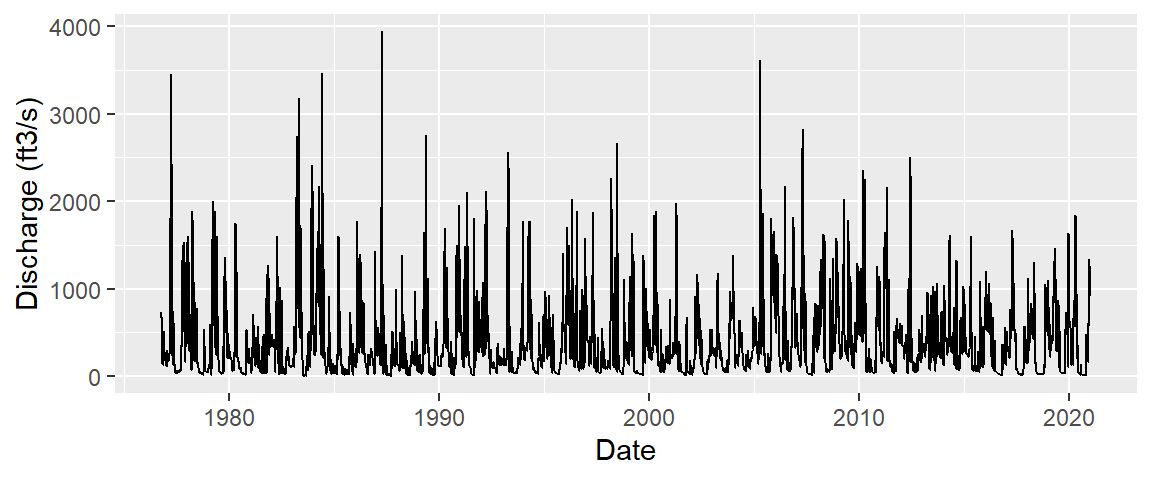The data exhibit a strong skew. We can check its distribution using a boxplot.

``ggplot(dat, aes(y = "", x = Discharge)) + geom_boxplot() + ylab("") + xlab("Discharge (ft3/s)")``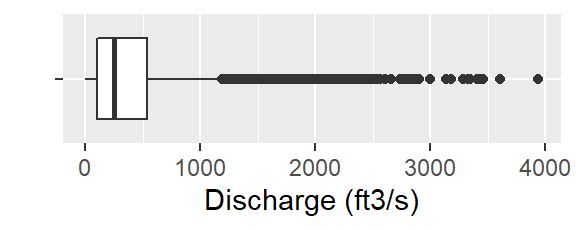A skewed data can bias the results of the analysis in favor of a small percentage of the observations while missing out on what the bulk of the data may be saying about the underlying process. A re-expression of the data is therefore strongly recommended.

## Re-expressing the data

A re-expression using a power of `1/5` seems to do a nice job in rendering a symmetrical distribution.

``````dat\$disp02 <- dat\$Discharge^(1/5)
ggplot(dat, aes(y = "", x = disp02)) + geom_boxplot() +
ylab("")  + xlab("Discharge (ft3/s)^0.2")``````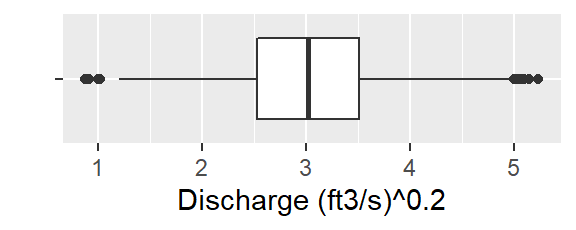Note that another way to plot the distribution is to keep the original values and scale the grid.

``````ggplot(dat, aes(y = "", x = Discharge)) + geom_boxplot() + ylab("")  + coord_trans(x = scales::boxcox_trans(1/5)) +
xlab("Discharge (ft3/s)")``````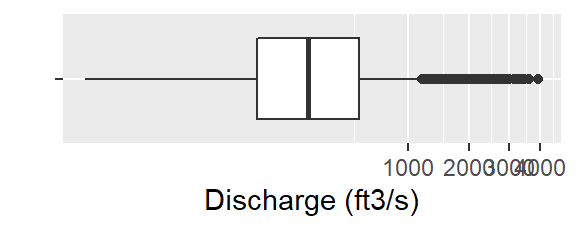Here’s a plot of the re-expressed discharge over time.

``````ggplot(dat, aes(Date, disp02)) + geom_line() +
ylab("Discharge (ft3/s)^0.2")``````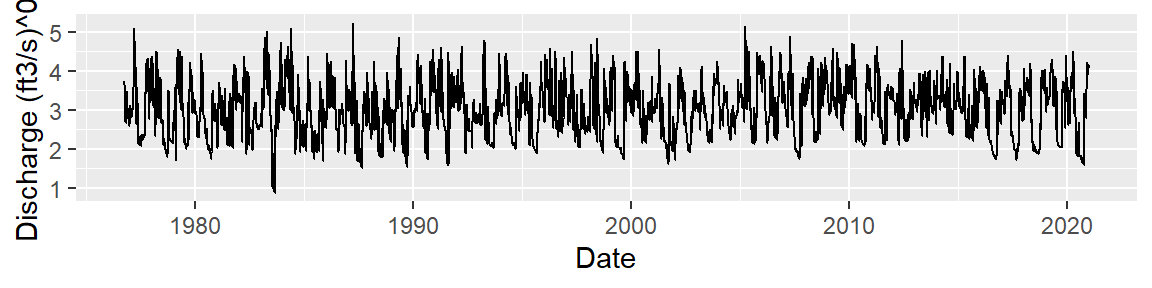## Exploring an overall trend

Given that an overall trend has a variability that seems much smaller than the total variability in the data, we’ll fit a loess to help guide our choice of a parametric model. We’ll plot both a traditional loess and a robust loess.

``````ggplot(dat, aes(Date, disp02)) + geom_line() +
ylab("Discharge (ft3/s)^0.2") +
geom_smooth(method = "loess", se = FALSE)+
geom_smooth(method = "loess", se = FALSE, col = "red",
method.args = list(family = "symmetric"))``````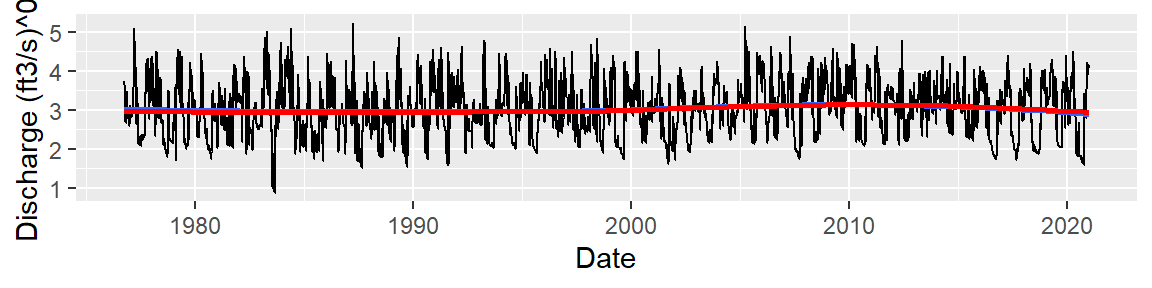There is no significant difference between the traditional loess (blue line) and the robust loess (red line) which suggest that any outlier in the data does not seem to wield a disproportionate influence on the model fit.

The smooths’ shape is difficult to make out given the range of y axis values. We’ll re-plot the data using a smaller y-axis range.

``````ggplot(dat, aes(Date, disp02)) + geom_line(alpha = 0.2) +
ylab("Discharge (ft3/s)^0.2") +
geom_smooth(method = "loess", se = FALSE) +
coord_cartesian(ylim = c(2.75, 3.25))``````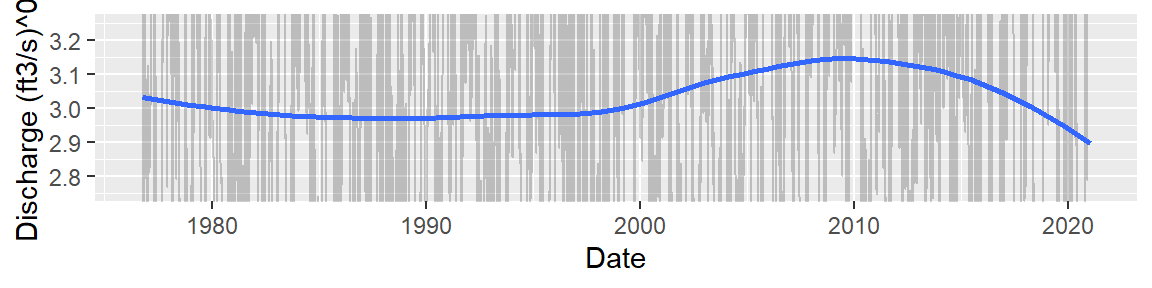Another approach to fitting a smooth to a noisy dataset is to aggregate the data across the range of x values. In this example, we summarize the discharge values by year. We’ll use the median as the summary statistic.

``````dat.sub <- dat %>%
mutate(year.int = as.integer(year(Date))) %>%
group_by(year.int) %>%
summarise(med = median(disp02, na.rm = TRUE))

ggplot(dat.sub, aes(year.int, med)) + geom_point() +
geom_smooth(method = "loess", se = FALSE, col = "blue") +
xlab("Year")``````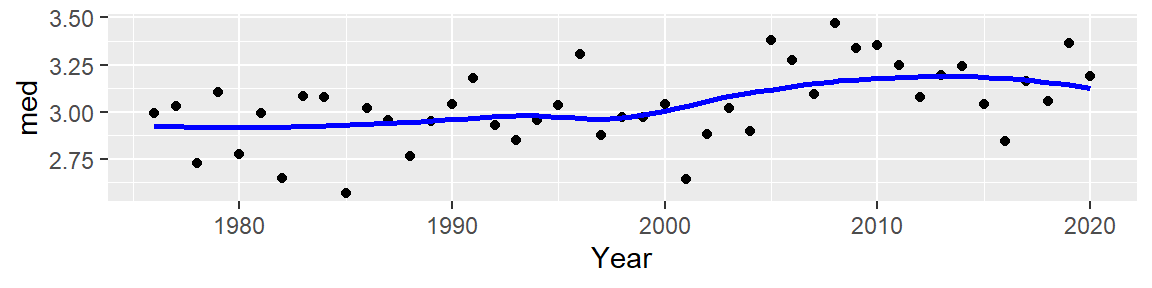In both cases, the patterns appear similar with a relatively linear discharge rate between the years 1975 and 1998 and a parabolic shape between 1998 and 2020.

There are at least two approaches that can be taken in modeling our observed trend when using a parametric mode: split the data into two parts and model each trend separately (i.e. pre and post 1998), or fit an overall model. We’ll adopt the latter. This will help us quantify an “overall” change in discharge even though the change may not be monotonic across all years using. We’ll fit a 1st order polynomial model using both a regular `lm` model and the `MASS` package’s robust `rlm` model.

``````ggplot(dat, aes(Date, disp02)) + geom_line(alpha = 0.2) +
ylab("Discharge (ft3/s)^0.2") +
geom_smooth(method = "lm", se = FALSE) +
geom_smooth(method = MASS::rlm, se = FALSE, col = "red")  +
coord_cartesian(ylim = c(2.5, 3.5))``````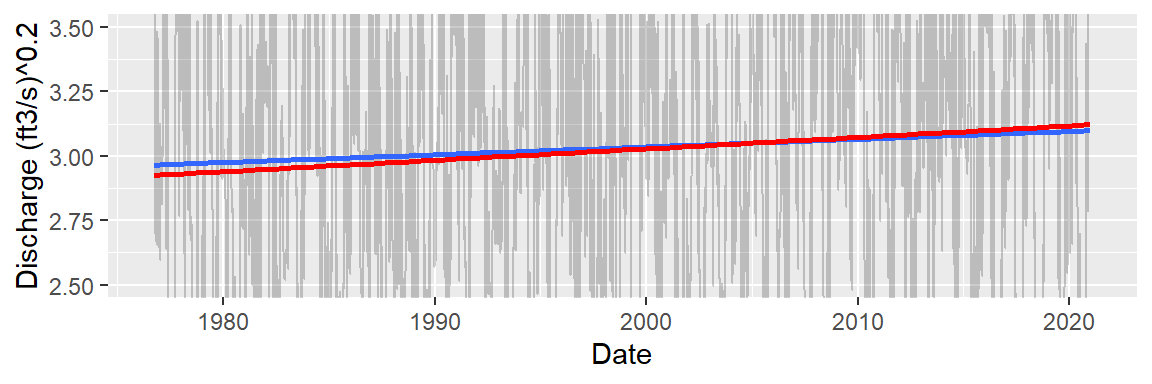The slopes between both the regular and robust regression models are different. This suggests that outliers in the data may be wielding a disproportionate influence on the data (this was not the case with the loess fit). We’ll adopt the robust model’s output in this analysis

``M1 <- MASS::rlm(disp02 ~ decimal_date(Date), dat)``

The modeled line is of the form \(Discharge^{1/5} = a + b(Year)\) with \(b\) = 0.0044 representing the average increase in discharge per year when expressed as a power of `0.2`. The overall trend (across all 44 years) explains about 0.20 (ft3/s)(0.2) of the variability in the data, or, when expressing the value back to the linear scale, 0.205 = 0.0003 ft3/s –a very small fraction of the overall variability in the data.

## Exploring the residuals

To properly explore the residuals for seasonal periodicity, we need to remove any multi-year patterns in the data. This is best performed using a loess. After exploring different loess spans, a span width of 0.3 was chosen. This is a smaller span that that used earlier when we modeled the overall trend (recall that we are seeking to remove multi-year patterns in this phase of the analysis).

``````ggplot(dat, aes(Date, disp02)) + geom_line(alpha = 0.2) +
ylab("Discharge (ft3/s)^0.2") +
geom_smooth(method = "loess", se = FALSE, span = 0.3) +
coord_cartesian(ylim = c(2.5, 3.5))``````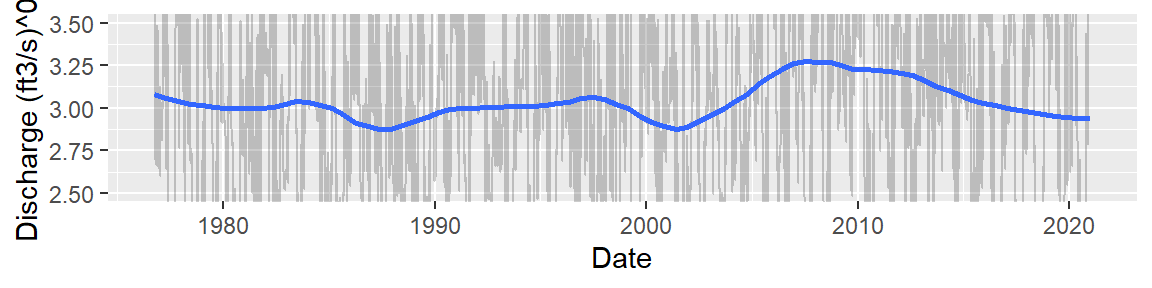``````L1 <- loess(disp02 ~ decimal_date(Date), dat, span = 0.3)
dat\$res1 <- L1\$residuals``````

The residual plot follows:

``````ggplot(dat, aes(Date, res1)) + geom_line() +
ylab("Residuals") ``````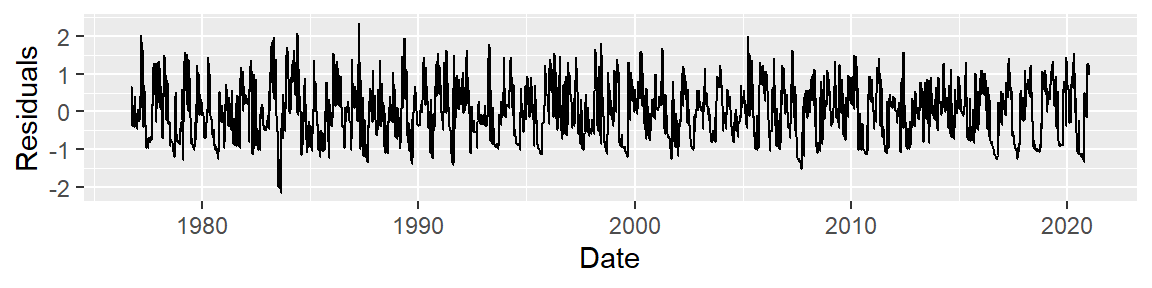## Exploring annual periodicity

The following plot groups the data by month of the year.

``````dat\$month <- month(dat\$Date, label = TRUE)

ggplot(dat, aes(month, res1)) + geom_boxplot() +
ylab("Residuals") ``````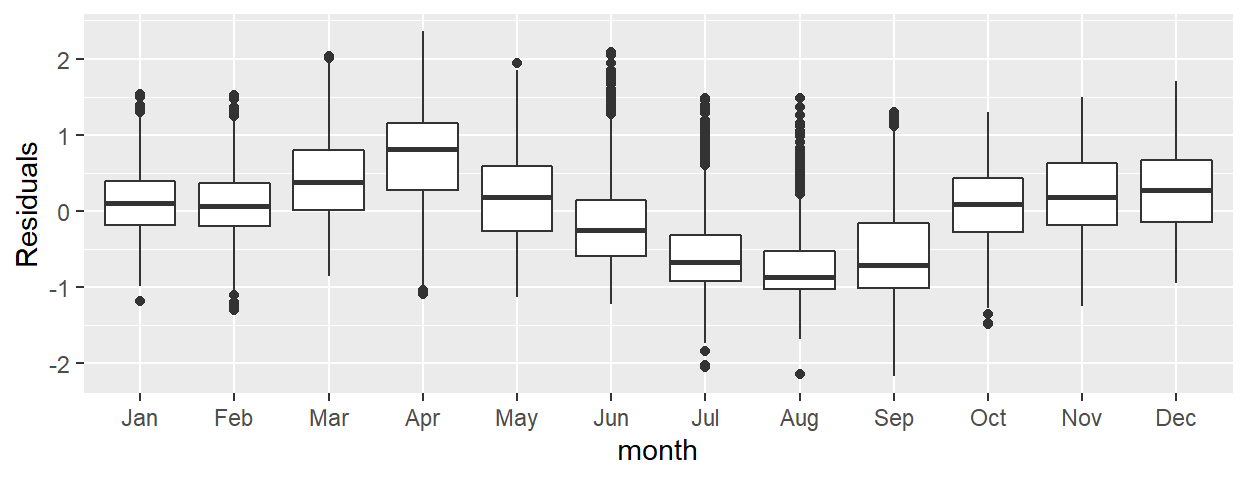A seasonal component can be clearly discerned from the above boxplots. From the plot, we can deduce that the seasonal component explains about 2.5 (ft3/s)(0.2) (or 2.55 ~ 100 ft3/s) of the variability in the data–a much bigger spread than that computed from the overall trend.